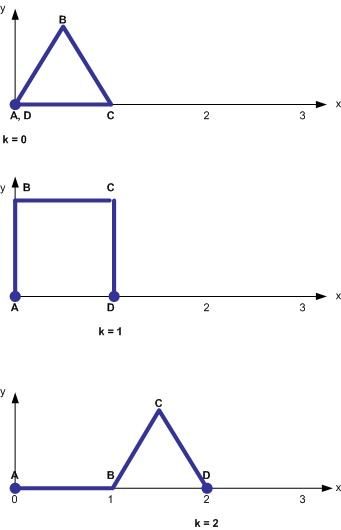## Mathematical Puzzle – Hinges

This is a puzzle.

Imagine I have three rods of length 1 each, and they are connected together with perfect hinges.  Together, they form a somewhat flexible shape ABCD (with AB=1, BC=1 and CD=1), even if I fix the two ends A and D to the floor at a given distance k apart.  The figure below shows examples for k=0, k=1, and k=2.For each of these, we assume that A is at (0,0) and that D is at (k,0).  For k=3, all flexibility disappears and there is only a single arrangement.  For any value of k, we can look at the relationship between the height of hinge B and the height of hinge C.  For some values of k, this relationship is simple; for others, the relationship is more involved.

For what values of k can you give a quantitative description of the relationship between the height of B and the height of C?  Can you sketch a graph, derive a formula, give an analysis?  I suggest you start with k=1, but don’t stop there!

This entry was posted in Uncategorized and tagged , , . Bookmark the permalink.

### 1 Response to Mathematical Puzzle – Hinges

1.Bert Speelpenning says:

What had me pose this puzzle is the disconnect between the simplicity of the scenario and the not-simplicity of the formal machinery we would normally bring to bear on the mathematical situation.
I could build this thing easily, with three length of straw with a string through it – the ends of the string taped to my desk. When I move the “hinge” up and down, the other hinge seems to know exactly where to go. There is no hesitation, no wondering what equation to apply, it just moves smoothly into position.
For k=0 and k=1, the shape and the movement is particularly familiar to us, and we can get our hands around what is going on, and describe it in easy terms.

For other values of k, we can approach this problem by introducing four variables that capture the position of B and C, set up three equations for the three lengths of the rods, and use two of the three equations to eliminate the horizontal coordinates of B and C, leaving a third equation only containing the two vertical coordinates of B and C. This equation gives a formal relationship between those two heights, even if it isn’t in the form where one height is expressed (by formula) as a function of the other.